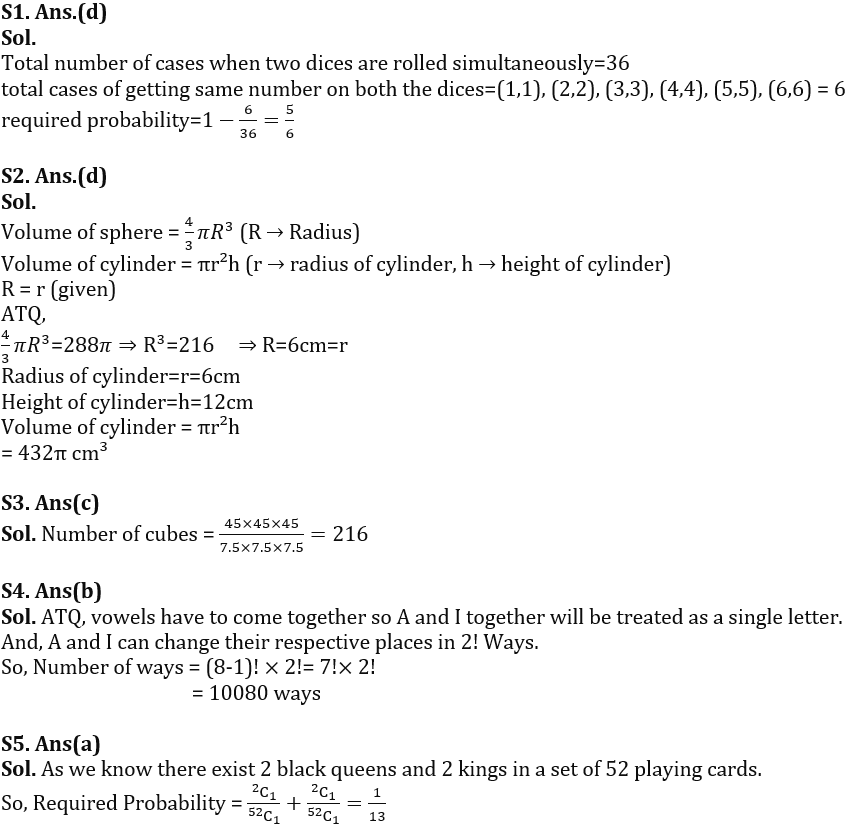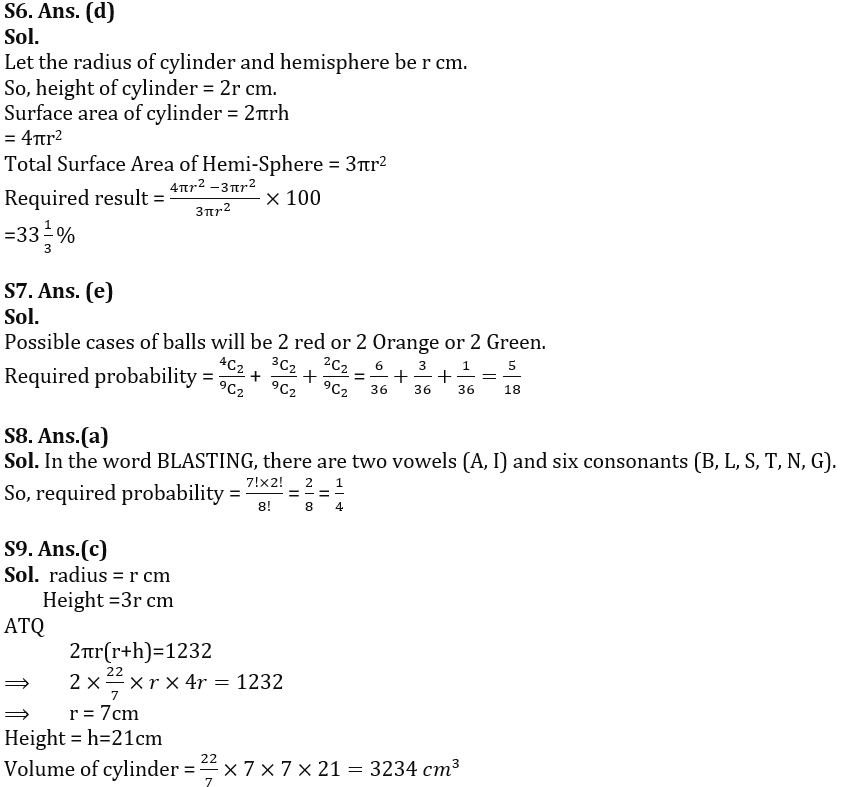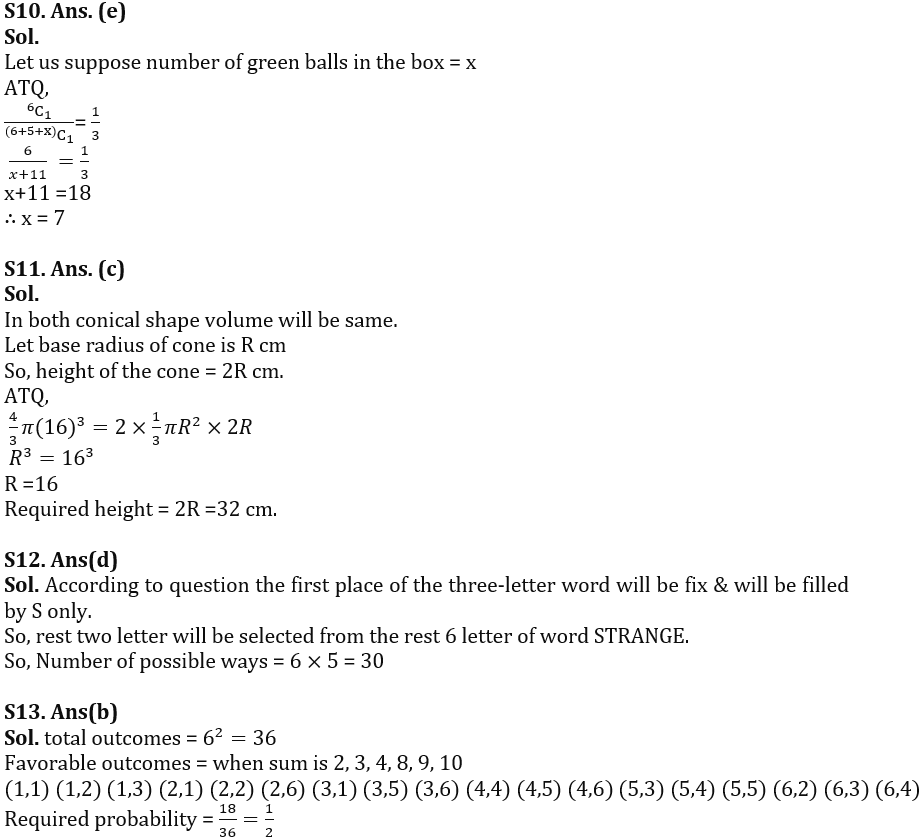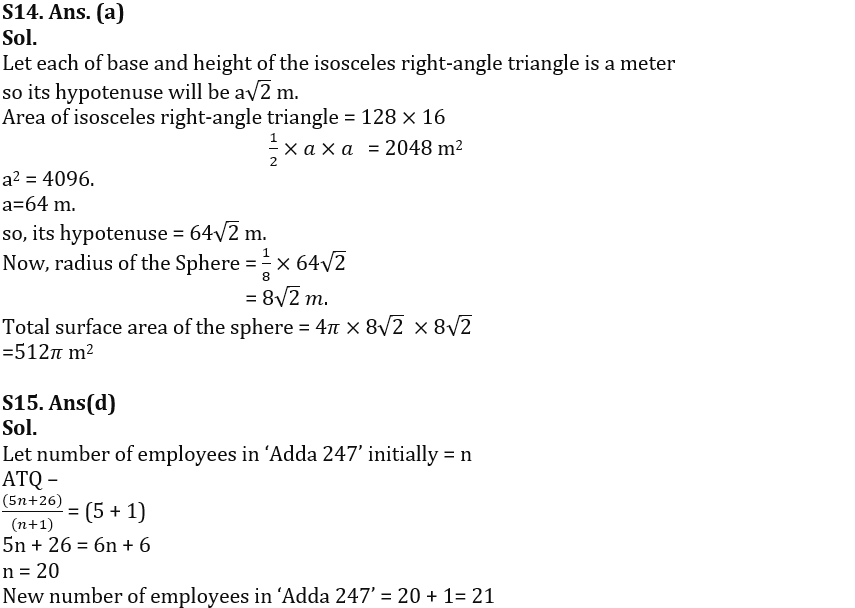Latest Banking jobs   »

# Quantitative Aptitude Quiz For IBPS RRB PO Prelims 2022- 23rd June

Q1. If two dices are rolled together, then find the probability of getting a number of one dice greater than the number on other dice?
(a) 3/4
(b) 2/3
(c) 1/6
(d) 5/6
(e) 1/2

Quantitative Quiz For IBPS RRB PO Prelims 2022 23rd June check in hindi

Q2. The radius of a cylinder & a sphere is same, and ratio of height and radius of cylinder is 2 : 1.If the volume of sphere is 288 π cm³ then find the volume of cylinder? (in cm³)
(a) 438 π
(b) 426 π
(c) 420 π
(d) 432 π
(e) 444 π

Q3. How many cubes of 7.5 cm edge can be cut out from a cube of 45 cm edge?
(a) 108
(b) 72
(c) 216
(d) 230
(e) 256

Q4. How many Words can be formed from the letters of the word ‘FLAGSHIP’ so that the vowels always come together?
(a) 5040
(b) 10080
(c) 720
(d) 360
(e) 1440

Q5. One card is picked randomly from a pack of 52 playing cards. What is the probability that it would either be black queen or red king?
(a) 1/13
(b) 5/13
(c) 6/13
(d) 7/13
(e) 8/13

Q6. The ratio of height of a cylinder to its base radius is 2:1 respectively. If radius of a hemisphere is equal to the radius of the cylinder, then find the total surface area of cylinder is what percent more than total surface area of a hemisphere?
(a) 40%
(b) 30%
(c) can’t be determined
(d) 33⅓%
(e) 50%

Q7. A bag contains 4 red, 3 orange and 2 green color balls. Find the probability of selecting two same color balls from the bag?
(a) 1/2
(b) 7/18
(c) 4/5
(d) 5/13
(e) 5/18

Q8. Find the probability of eight letters word that can be formed from the letters of the word ‘BLASTING’ so that vowels always come together.
(a) 1/4
(b) 2/5
(c) 1/3
(d) 10/21
(e) 5/14

Q9. The total surface area of a cylindrical vessel is 1232 cm² and the height of vessel is 2 times more than the radius of vessel. Find the volume of cylindrical vessel?
(a) 4312 cm³
(b) 3201 cm³
(c) 3234 cm³
(d) 3256 cm³
(e) 3333 cm³

Q10. There are 5 red balls, 6 black balls and some green colored balls in a box. If the probability of choosing a black ball from the box is ⅓, then find the number of green-colored ball in the box?
(a) 5
(b) 4
(c) 6
(d) 8
(e) 7

Q11. A spherical ball of radius 16 cm is melted and casted into two cones of equal size and shape. If the base radius of the cone is 50% of the height of the cone. Find the height of each cone?
(a) 36 cm
(b) 18 cm
(c) 32 cm
(d) 20 cm
(e) 16 cm

Q12. How many three letters words starting with S (with or without meaning) can be formed out of the letters of the word, “STRANGE”, if repetition of letters is not allowed?
(a) 10
(b) 15
(c) 12
(d) 30
(e) 18

Q13. If two dice are rolled simultaneously, find the probability of obtaining the sum (of numbers on these two dices) which is divisible by 2 or 3 but not by both?
(a)1/4
(b) 1/2
(c) 1/5
(d) 1/6
(e) 1/3

Q14. The area of a rectangular field having length 128m and breadth 16m is equal to the area of an isosceles right-angle triangle. If the radius of a sphere is 12 ½ % of the hypotenuse of the isosceles right-angle triangle, then find out the total surface area of sphere?
(a) 512π m²
(b) 343π m²
(c) 580π m²
(d) 494π m²
(e) 500π m²

Q15. Gurdeep Chhabra joined ‘Adda 247’ with the work experience of 26 years due to which average work experience of all employees of ‘Adda 247’ was increased by one year. If initial average work experience of all employees of ‘Adda 247’ was five years, then find the new number of employees in ‘Adda 247’?
(a) 23
(b) 19
(c) 25
(d) 21
(e) 27

Solutions#### Congratulations!